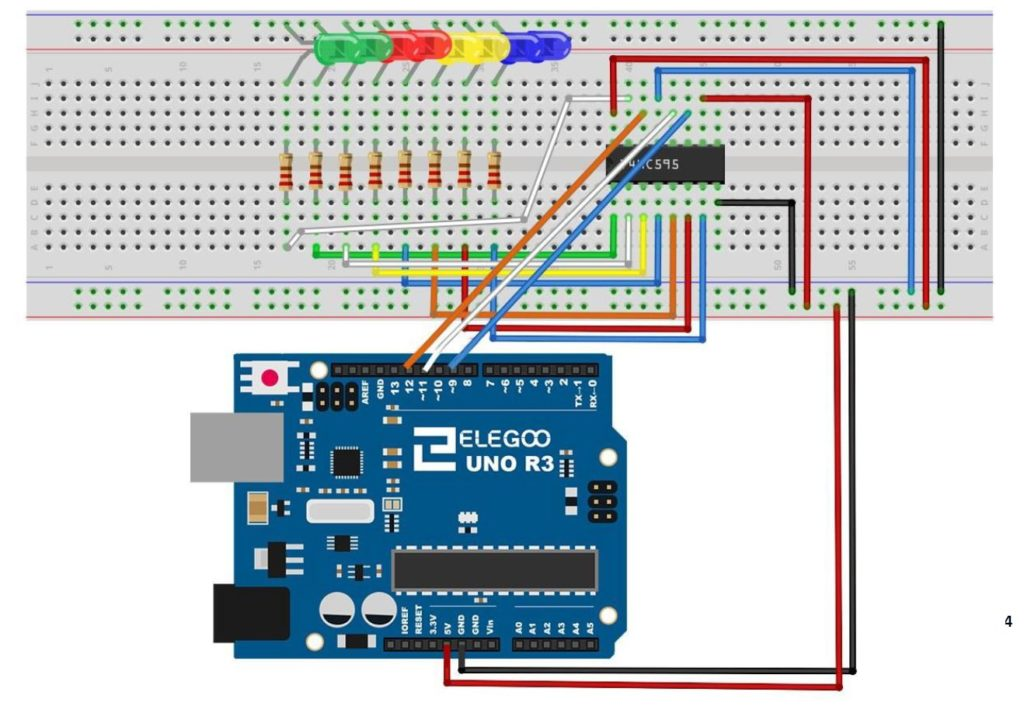# Arduino Tutorial 44: Understanding Logical Shift Left and Logical Shift Right with the74HC595

In this lesson, we explore how to perform Logical Shift Left (LSL), and Logical Shift Right (LSR) functions on binary numbers, and we implement a circuit to perform these functions using an Arduino and a 74HC595 chip. We will demonstrate these functions on 8 bit binary numbers.

We start with the basic circuit and code which were developed in Lesson 42. In this lesson we are using parts from the Elegoo Arduino kit, which you can get  HERE.  We start with this circuit, which was explained in Lesson 42.This is the schematic we use in this example to control 8 LEDs from the 74HC595 chip.

You can see that with this circuit, an 8 bit binary number can be visually displayed by illuminating the circuit LED. The goal of this lesson is to write code to perform LSL and LSR functions. The graphics below show conceptually how simple these functions are:

MSB stands for “Most Significant Bit” and LSB stands for “Least Significant Bit”.  You can see that the LSL function just moves each bit one to the left, and fills the empty LSB with a “0”.

The LSR funtion is just as simple as illustrated below.

Such shifts are often required when doing digital logic, so it is important to understand what the terms mean.

We can see that the LSL function can be performed by simply multiplying the binary number by 2. Similarly the LSR function can be performed by dividing the binary number by 2.

Code for LSL:

Code for LSR function:

# Arduino Tutorial 43: Binary Counter with 74HC595 Serial to Parallel Shift Register

In lesson 42 we showed you how to connect and program the 74HC595 shift register. We showed how data in byte format would then be written to an array of 8 LED to give a visual representation of the binary version of that byte variable. We then gave you the assignment to create a Binary Counter using the 4HC595. In this lesson we show you the solution. This builds on Lesson 42, so make sure to have your basic 74HC595 circuit set up before starting this lesson.

In this lesson we are using parts from the Elegoo Arduino kit, which you can get  HERE.

The code we ended up developing in this lesson is provided below.